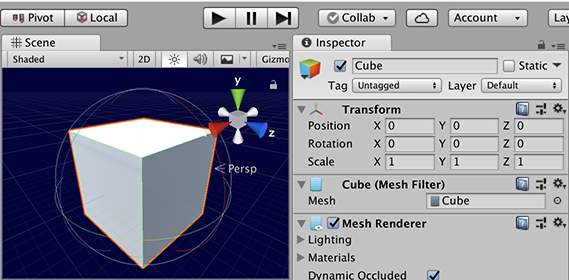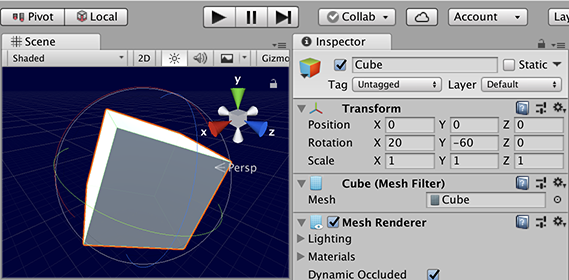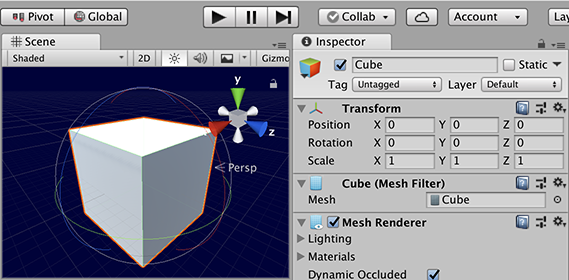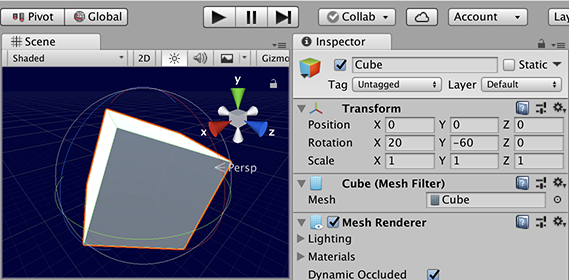Version: 2019.4

# Transform.Rotate

マニュアルに切り替える

## 説明

Use Transform.Rotate to rotate GameObjects in a variety of ways. The rotation is often provided as an Euler angle and not a Quaternion.

You can specify a rotation in world axes or local axes.

World axis rotation uses the coordinate system of the `Scene`, so when you start rotate a GameObject, its x, y, and z axes are aligned with the x, y, and z world axes. So if you rotate a cube in world space, its axes align with the world. When you select a cube in the Unity Editor’s `Scene` view, rotation `Gizmos` appear for the left/right, up/down and forward/back rotation axes. Moving these `Gizmos` rotates the cube around the axes. If you de-select and then re-select the cube, the axes restart in world alignment.

Local rotation uses the coordinate system of the GameObject itself. So, a newly created cube uses its x, y, and z axis set to zero rotation. Rotating the cube updates the rotation axes. If you de-select and the re-select the cube, the axes are shown in the same orientation as before.A cube not rotated in Local Gizmo ToggleA cube rotated in Local Gizmo ToggleA cube not rotated in Global Gizmo ToggleA cube rotated in Global Gizmo Toggle

For more information on Rotation in Unity, see Rotation and Orientation in Unity.

public void Rotate (Vector3 eulers, Space relativeTo= Space.Self);

## パラメーター

 eulers The rotation to apply. relativeTo Determines whether to rotate the GameObject either locally to the GameObject or relative to the Scene in world space.

## 説明

Applies a rotation of eulerAngles.z degrees around the z-axis, eulerAngles.x degrees around the x-axis, and eulerAngles.y degrees around the y-axis (in that order).

Rotate takes a Vector3 argument as an Euler angle. The second argument is the rotation axes, which can be set to local axis (Space.Self) or global axis (Space.World). The rotation is by the Euler amount.

public void Rotate (float xAngle, float yAngle, float zAngle, Space relativeTo= Space.Self);

## パラメーター

 relativeTo Determines whether to rotate the GameObject either locally to the GameObject or relative to the `Scene` in world space. xAngle Degrees to rotate the GameObject around the X axis. yAngle Degrees to rotate the GameObject around the Y axis. zAngle Degrees to rotate the GameObject around the Z axis.

## 説明

The implementation of this method applies a rotation of `zAngle` degrees around the z axis, `xAngle` degrees around the x axis, and `yAngle` degrees around the y axis (in that order).

Rotate can have the euler angle specified in 3 floats for x, y, and z.

The example shows two cubes: one cube uses Space.Self (the local space and axes of the GameObject) and the other uses Space.World (the space and axes in relation to the /Scene/). They are both first rotated 90 in the X axis so they are not aligned with the world axis by default. Use the xAngle, yAngle and zAngle values exposed in the inspector to see how different rotation values apply to both cubes. You might notice the way the cubes visually rotate is dependant on the current orientation and Space option used. Play around with the values while selecting the cubes in the scene view to try to understand how the values interact.

```using UnityEngine;// Transform.Rotate example
//
// This script creates two different cubes: one red which is rotated using Space.Self; one green which is rotated using Space.World.
// Add it onto any GameObject in a scene and hit play to see it run. The rotation is controlled using xAngle, yAngle and zAngle, modifiable on the inspector.public class ExampleScript : MonoBehaviour
{
public float xAngle, yAngle, zAngle;    private GameObject cube1, cube2;    void Awake()
{
cube1 = GameObject.CreatePrimitive(PrimitiveType.Cube);
cube1.transform.position = new Vector3(0.75f, 0.0f, 0.0f);
cube1.transform.Rotate(90.0f, 0.0f, 0.0f, Space.Self);
cube1.GetComponent<Renderer>().material.color = Color.red;
cube1.name = "Self";        cube2 = GameObject.CreatePrimitive(PrimitiveType.Cube);
cube2.transform.position = new Vector3(-0.75f, 0.0f, 0.0f);
cube2.transform.Rotate(90.0f, 0.0f, 0.0f, Space.World);
cube2.GetComponent<Renderer>().material.color = Color.green;
cube2.name = "World";
}    void Update()
{
cube1.transform.Rotate(xAngle, yAngle, zAngle, Space.Self);
cube2.transform.Rotate(xAngle, yAngle, zAngle, Space.World);
}
}
```

public void Rotate (Vector3 axis, float angle, Space relativeTo= Space.Self);

## パラメーター

 angle The degrees of rotation to apply. axis The axis to apply rotation to. relativeTo Determines whether to rotate the GameObject either locally to the GameObject or relative to the Scene in world space.

## 説明

Rotates the object around the given axis by the number of degrees defined by the given angle.

Rotate has an axis, angle and the local or global parameters. The rotation axis can be in any direction.